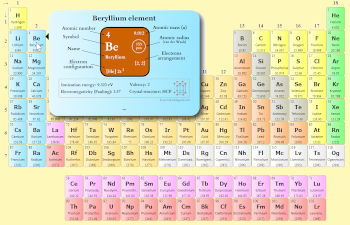# 2 Reasons Why Periodic table is Divided into Blocks (Simple)

The reasons why periodic table is divided into blocks are given below:

• Because blocks represent the orbitals (or subshells) in which the valence electrons are present.
• By knowing the position of elements in the block, you can easily find the number of orbits (shells) of an atom.

I know you haven’t understood these above reasons.

So I have explained them below one by one.

## Reason #1: Because blocks represent the orbitals (or subshells) in which the valence electrons are present

First of all you might know that there are 4 blocks on periodic table

They are;

• s-block
• p-block
• d-block
• f-block

You might be knowing about the shells around the nucleus of an atom.

Shells are also known as orbits.

For example, magnesium has 3 orbits (or 3 shells).

Now the question is,

What are orbitals? And what does s, p, d and f represent?

Orbitals are the space around the nucleus, where the probability of finding the electrons is maximum.

And s, p, d and f represents the regions around the nucleus of an atom.

These regions around the nucleus are known orbitals (or subshells.)

Now the s-block includes the elements of group 1 and group 2.

This means that these elements have the valence electrons in the s-subshell (or s-orbital.)

Similarly, p-block includes the elements from group 13 to group 18.

Elements lying in the p block indicate that these elements have their valence electrons in p subshells (or p-orbitals.)

Similarly the elements lying in group 3 to group 12 are included in d block.

Hence their valence electrons are in d-orbitals.

And the last block which is at the bottom of the modern periodic table is the f-block.

The elements in the f-block indicate that they have the valence electrons in the f orbitals (or f subshells.)See how this Interactive Periodic Table helps you

• You can effortlessly find every single detail about the elements from this single Interactive Periodic table.
• You will get the detailed information about the periodic table which will convert a newbie into pro.
• You will also get the HD images of the Periodic table (for FREE).

Visit ➢ Periodic table

## Reason #2: By knowing the position of elements in the block, you can easily find the number of orbits (shells) of an atom

Let me ask you a question,

How many orbits does potassium have? And in which orbitals its valence electrons are?

At this moment you do not know the answer of this question.

But if you know the position of an element, you can easily answer this question.

In the Periodic table, the potassium element lies in the s-block.

Hence, first thing is cleared that it has the valence electrons in s-orbitals.

Now the question is, how many orbits does potassium have?

So for that we have to see the position of an element in the block.

Here the potassium element lies at the 4th position (or 4th period), hence it has 4 orbits.

This way, you can easily find the orbits as well as orbitals by knowing the position of elements in the  blocks.